MATLAB Function Referencebetainc

Incomplete beta function

Syntax

• ```I = betainc(X`,`Z,W)
```

Definition

The incomplete beta function is

•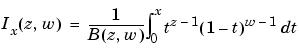where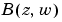, the beta function, is defined as

•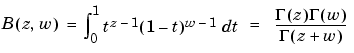and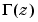is the gamma function.

Description

```I = betainc(X,Z,W) ``` computes the incomplete beta function for corresponding elements of the arrays `X`, `Z` and `W`. The elements of `X` must be in the closed interval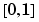. The arrays `Z` and `W` must be nonnegative and real. All arrays must be the same size, or any of them can be scalar.

Examples

• ```format long
betainc(.5,(0:10)',3)

ans =
1.00000000000000
0.87500000000000
0.68750000000000
0.50000000000000
0.34375000000000
0.22656250000000
0.14453125000000
0.08984375000000
0.05468750000000
0.03271484375000
0.01928710937500
```

See Also

`beta`, `betaln`

© 1994-2005 The MathWorks, Inc.